# Inter Maths solutions for Hyperbola

Inter Maths IIB solutions for Hyperbola exercise 5(a) are given.

These solutions are very easy to understand. First you study the textbook lesson very well.

Observe the example problems and solutions given in the textbook. Try them.

Observe the solutions given below and try them in your own method.

You can also see the solutions

SSC Maths text book Solutions class 10

Inter maths 1A textbook solutions

Inter Maths 1B textbook solutions

Inter Maths 1IA text book solutions

Inter Maths IIB text book solutions

M

# Inter Maths 2B solutions for Hyperbola

Exercise 5(a)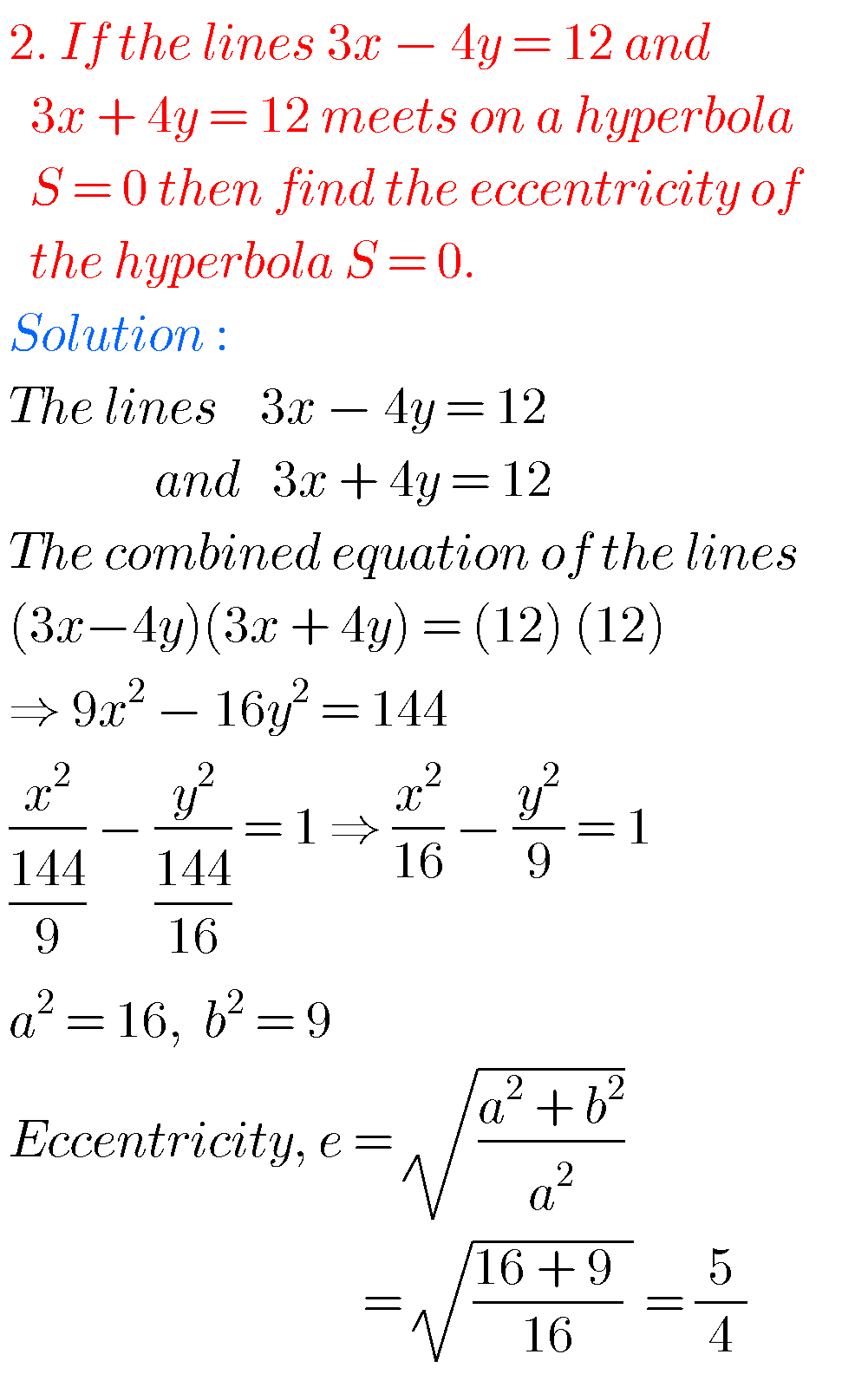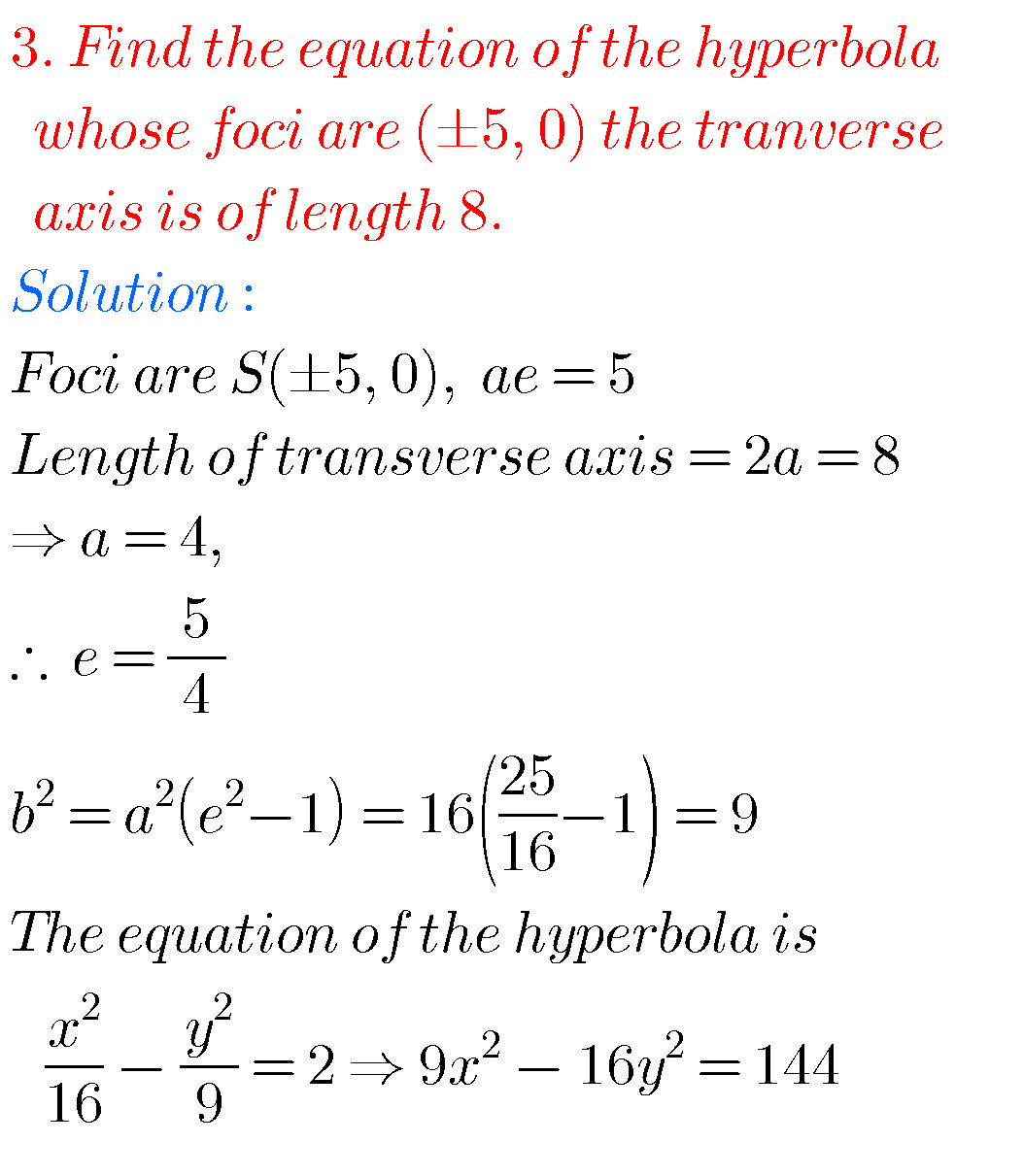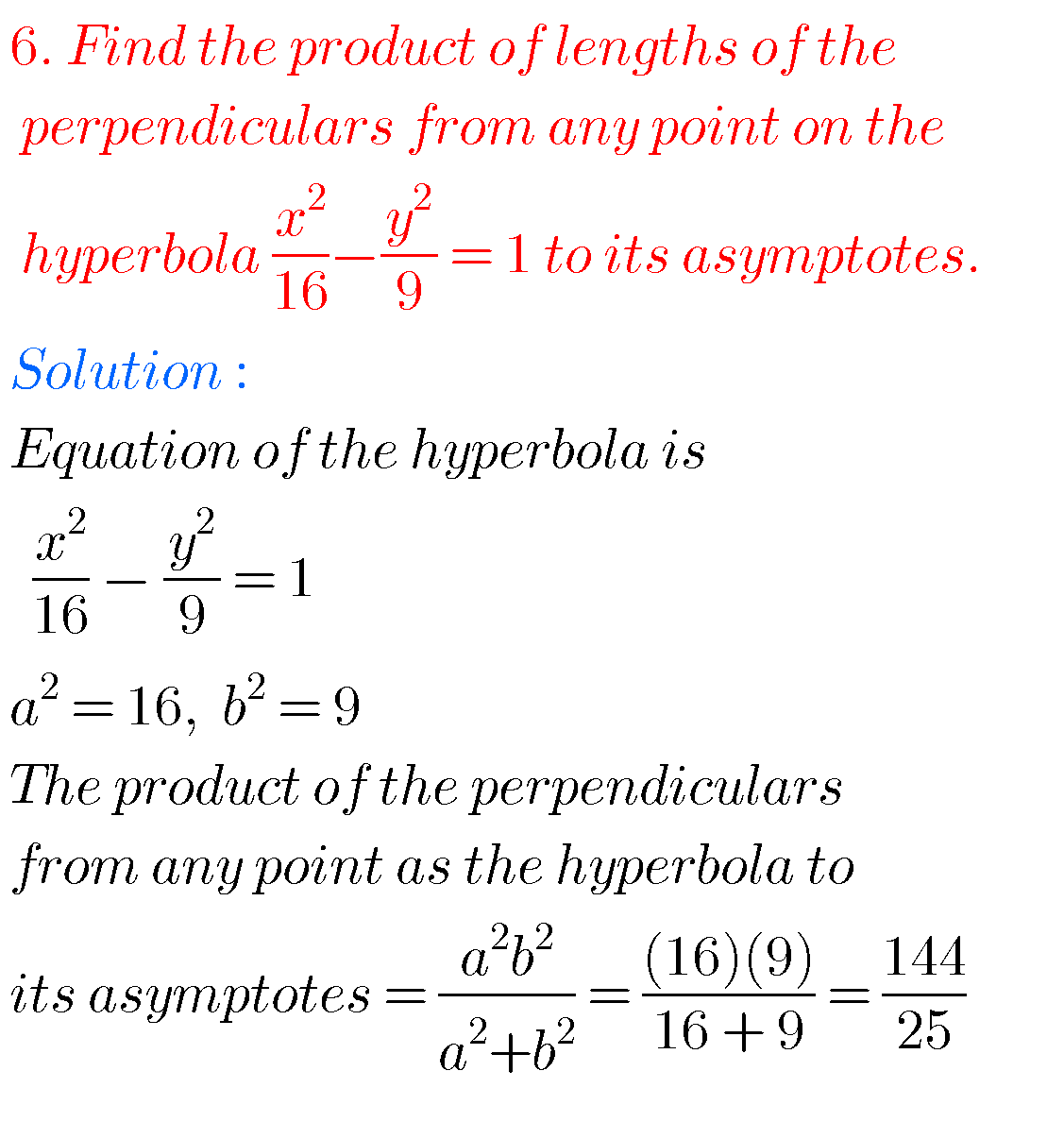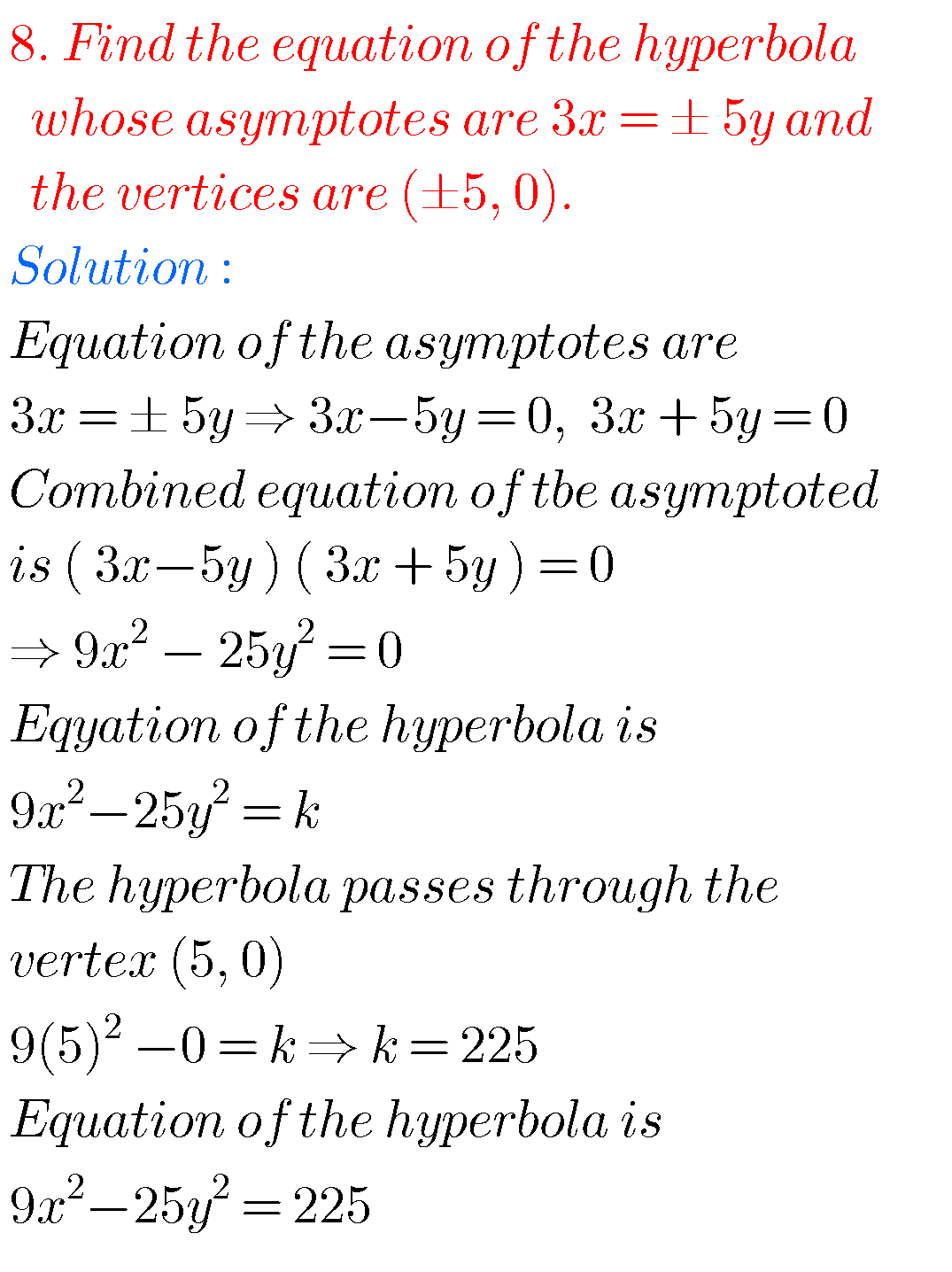## Maths IIB Inter solutions for Hyperbola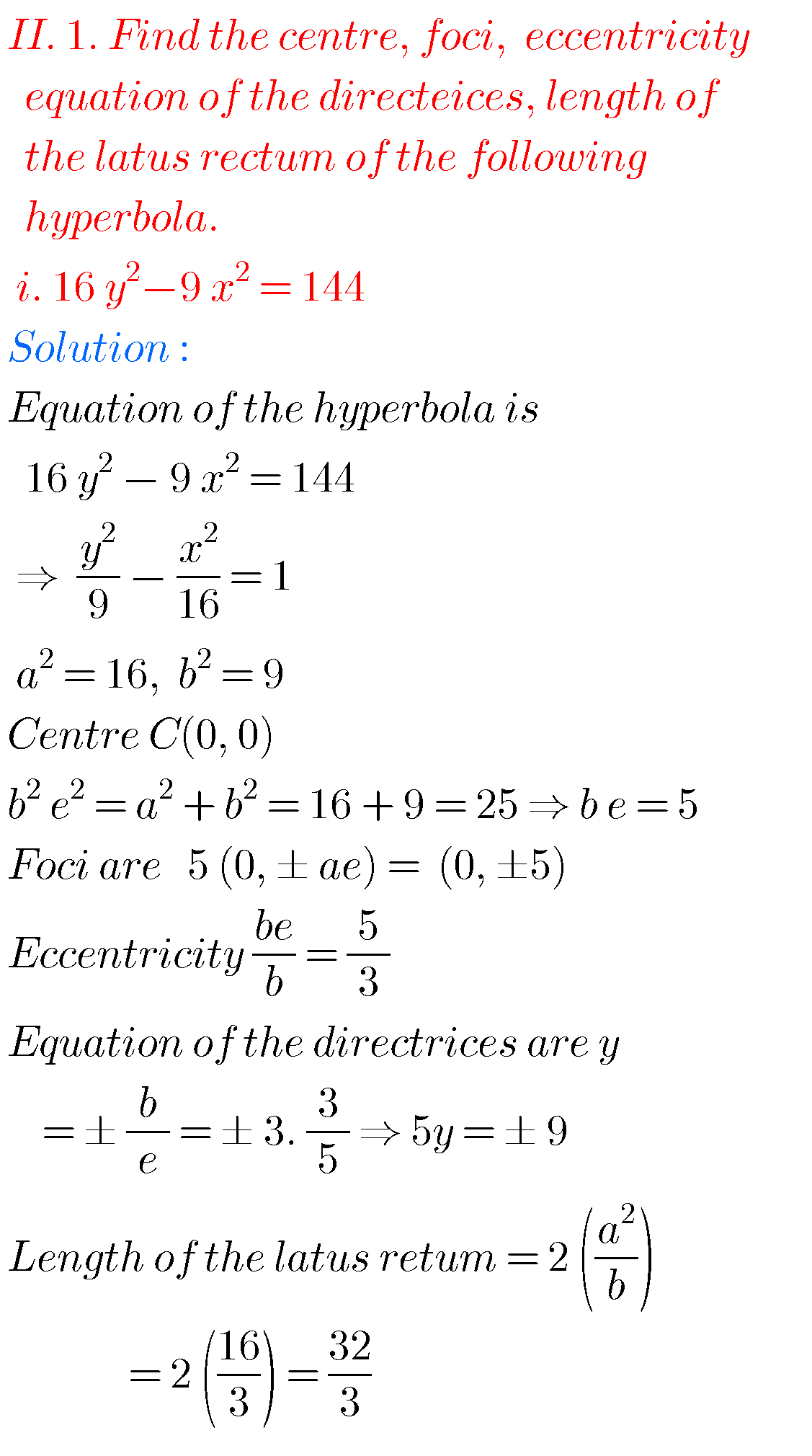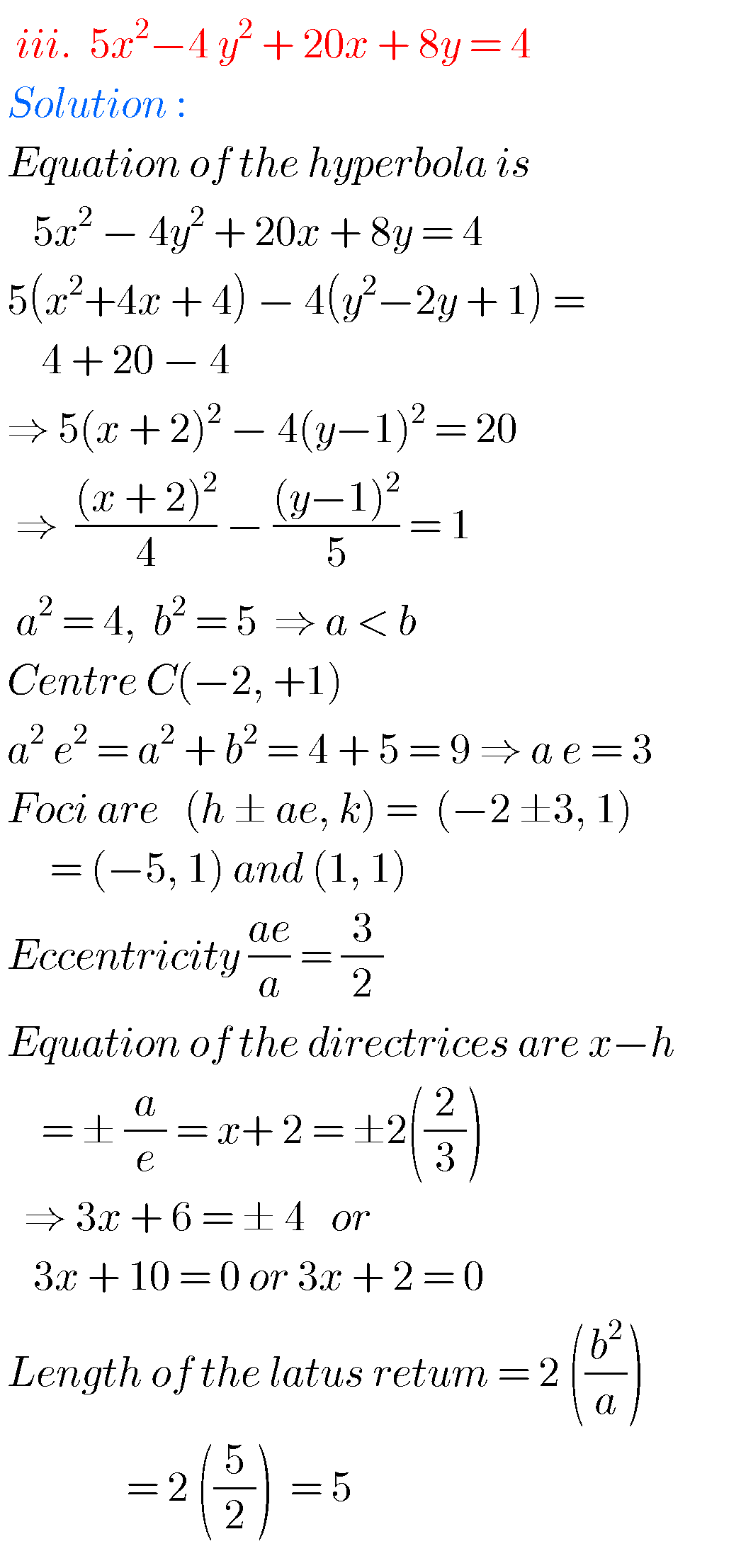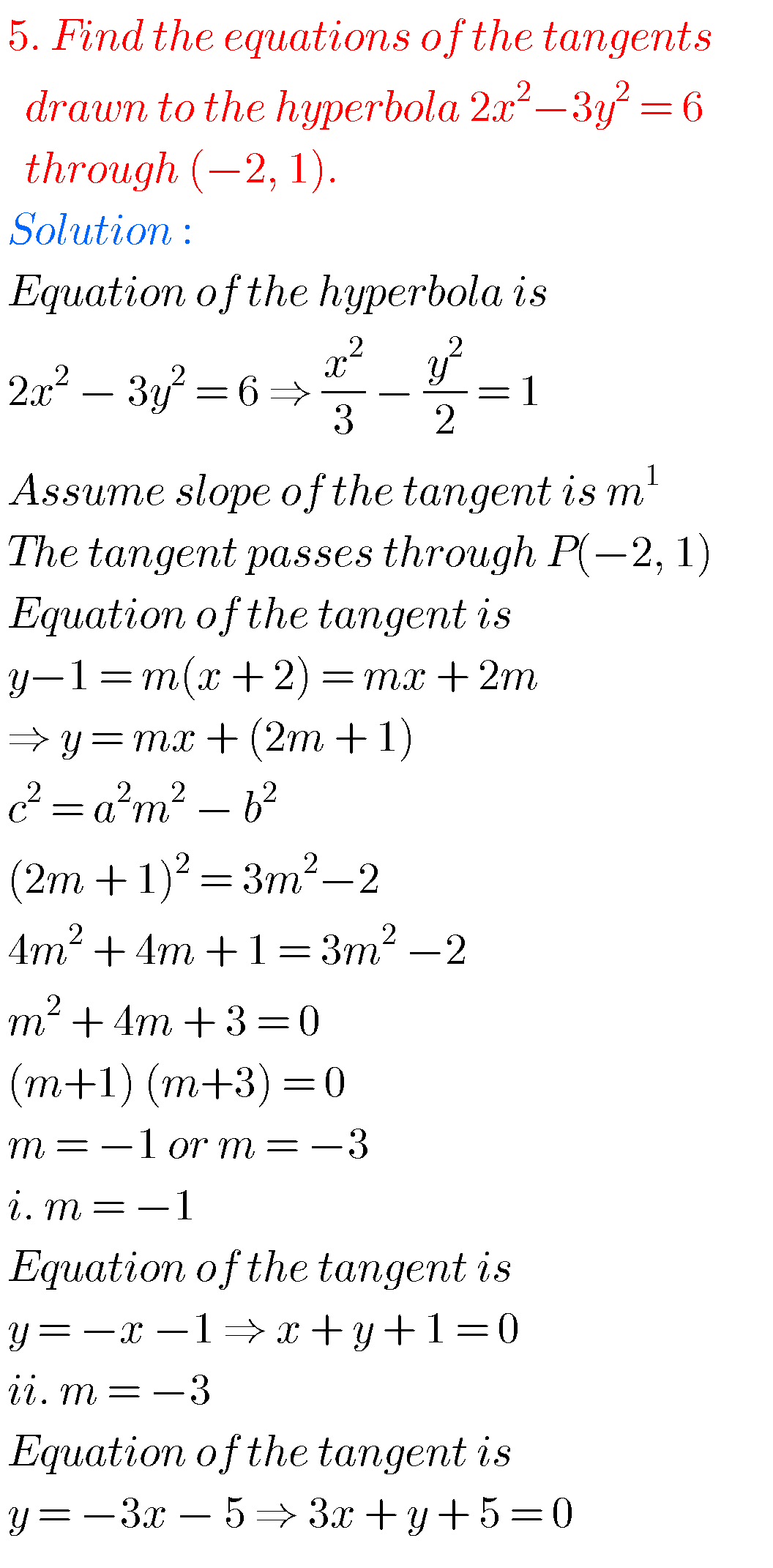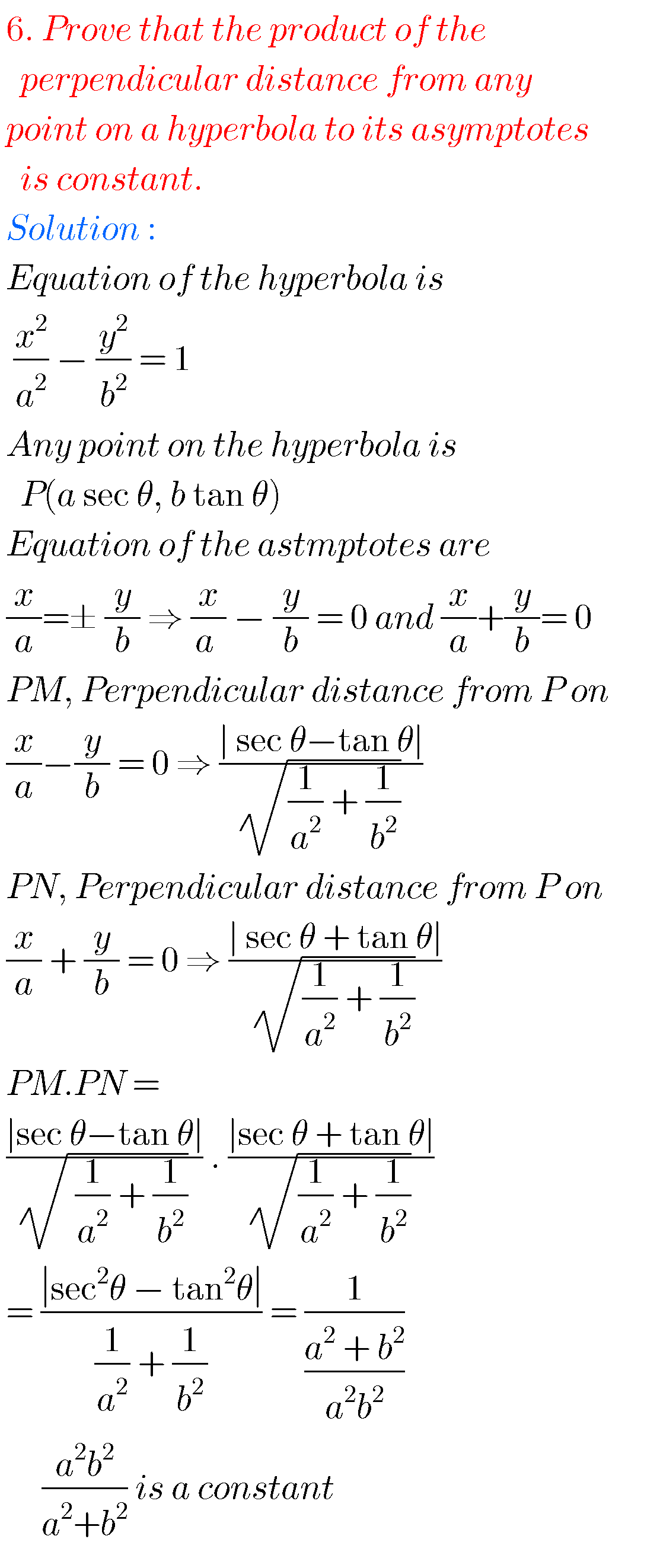### Hyperbola solutions Inter Maths 2bNote : Observe the solutions and try them in your own method.